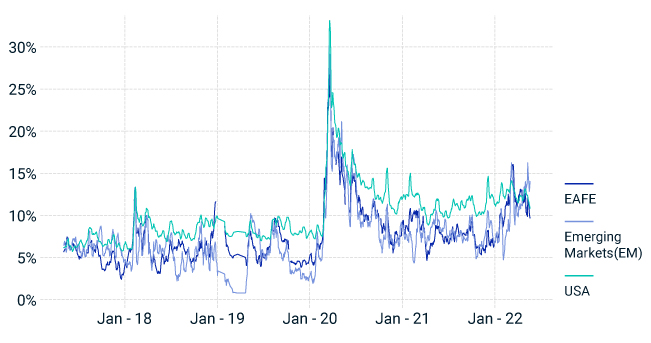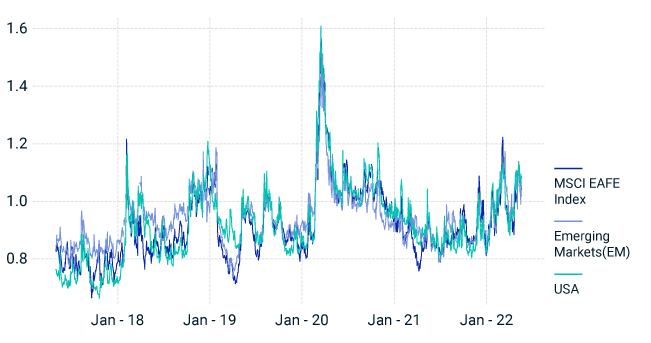## Index Options Indicated Negative Market Sentiment Intro

May 25, 2022

In the face of continued global equity-market volatility, we turn to the listed-options market to gauge the perception of market risk over the coming months for EAFE, emerging markets (EM) and the U.S.1

The four options-based indicators we analyzed showed investors were concerned about the trajectory of equity markets.

1. Heightened levels of implied volatility

Three-month implied volatility across regions remained elevated, with levels in the top decile, 91st percentile, for EAFE and the U.S., and around the 83rd percentile for EM, based on 5-years of history.2. For the U.S., the current levels stood at 88th percentile based on data since January 2000. (See chart one.)

2. Steep implied-volatility skew: higher premiums for puts vs. calls

We observed rising skew across regions with heightened negative sentiment for EM relative to the U.S. and EAFE. Skew stood at 14% (93nd percentile) for EM; the U.S. and EAFE stood at 11% and 9% respectively (62nd percentile for the U.S. and 76th percentile for EAFE). (See chart two.)

3. Inverted-term structure: Short-dated volatility was higher than longer dated volatility

The term structure calculated using 91- to 365-day implied-volatility ratio of at-the-money (ATM) options remained inverted (ratio above 1), indicating higher short-term vs. long-term volatility. (See chart three.)

4. High CBOE Total Put/Call Ratio: Put volumes outpaced call volumes

A high CBOE Total Put/Call Ratio (calculated using index and equity options) suggests bearish sentiment. The measure stood at 1.1 based on a 10-day moving average, as of May 20, 2022. Over the last 10 years, the ratio has risen above this level only four times: March 2020, December 2018, November 2016 and September 2015.

#### Average implied volatilityImplied volatility is based on the average implied volatility of 91-day ATM call and put options from May 1, 2017 to May 20, 2022. Source: Optionmetrics.

#### Implied volatility skewImplied-volatility skew is calculated using the five-day moving average of the put-call implied-volatility spread of the 91-day 20-delta strikes, from May 1, 2017, to May, 20, 2022. Source: OptionMetrics

#### Volatility term structureTerm Structure is calculated using 91-day to 365-day implied volatility ratio of ATM options, from May 1, 2017, to May 20, 2022. Source: OptionMetrics

1Options linked to the MSCI EAFE, MSCI Emerging Market and S&P 500 Indexes have been used for the EAFE, EM and U.S. implied volatility calculations, respectively.

2Percentiles calculated based on data since May 1, 2017.